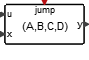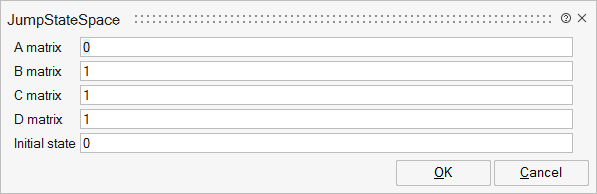# JumpStateSpace

This block implements a continuous-time linear state-space system with the possibility of jumps in the state. The first input is the regular input signal of the linear system, the second carries the new value of the state which is copied into the state when an event activates the block through its unique activation input port.Dynamical

## Description

The JumpStateSpace block realizes a continuous-time, linear state-space system with the possibility of jumps in the state. The block has two inputs: The first input is the regular input of the linear system; the second carries the new value of the state which is copied into the state when an event arrives at the block's unique input port, meaning that the state of the system jumps to the value present on the second input (of size equal to that of the state). The system is defined by the (A,B,C,D) matrices and the initial state x0. The dimensions must be compatible. The sizes of inputs and outputs are adjusted automatically.

## ParametersNameLabelDescriptionData TypeValid Values

A

A matrix

real nxn matrix where n is the number of states, default value: 1.

Matrix

B

B matrix

real nxm matrix where n is the number of states and m is the number of inputs, default value: 1. B=[ ] if block has no input.

Matrix

C

C matrix

real rxn matrix, default value: 1. r is the number of outputs and n is the number of states.

Matrix

D

D matrix

real rxm matrix where r is the number of outputs and m is the number of inputs, default value: 1.

Matrix

x0

Initial state

vector of size n or scalar (expanded to a vector of size n).

Vector

## Ports

NameTypeDescriptionIO TypeNumber

y

explicit

output

1

u

explicit

input

1

x

explicit

input

2

jump

activation

input

1

NameValueDescription

always active

yes

direct-feedthrough

no

The first input is direct feedthrough unless D is zero. The second input is always direct feedthrough

zero-crossing

no

mode

no

continuous-time state

yes

discrete-time state

no